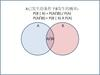1763年《机会学说中一个问题的解》

P(A|B)=P(B|A)*P(A)/P(B)

Thomas Bayes

Bayes Rule

• 2022-03-24 19:45:55

# 贝叶斯公式

## 引言

曲奇饼问题：假设有两碗饼干，碗1中有30个香草饼干和10个巧克力饼干，碗2中含有香草和巧克力饼干各20个。随机的从一个碗中拿一块饼干，拿到的饼干是香草味的，问：这个香草饼干是从碗1中拿到的概率是多少？

事件A：饼干是在碗1中拿的；

事件B：拿到饼干是香草饼干；

显然事件A的先验概率 P ( A ) = 1 2 P(A)=\frac{1}{2} ，可以将已知拿到的饼干是香草饼干(即事件B)理解为证据，在知道这个证据后，对事件A发生的概率做一个重新评估，即事件A的后验概率 P ( A ∣ B ) P(A|B)

## 贝叶斯公式的理解

如下为贝叶斯公式，其中 P ( A ) P(A) 为事件A的先验概率 P ( A ∣ B ) P(A|B) 为事件 A 的后验概率 ，且后验概率的计算融合了先验概率的值。
P ( A ∣ B ) = P ( A ) P ( B ∣ A ) P ( B ) P(A|B)=\frac{P(A)P(B|A)}{P(B)}
这里事件B可以理解为证据，事件 A 可以理解为待推理的事件，后验概率 P ( A ∣ B ) P(A|B) 是在给出事件 B 为证据的基础上，对 P ( A ) P(A) 值的推理更新。

对贝叶斯公式的理解：后验概率 P ( A ∣ B ) P(A|B) 就是先验概率 P ( A ) P(A) 乘以一个调整因子 P ( B ∣ A ) P ( B ) \frac{P(B|A)}{P(B)} ，亦即根据数据或信息的更新，对事件的可能性进行重新评估。

## 贝叶斯公式的推导

在推到贝叶斯公式之前，首先需要了解条件概率公式：
P ( A ∣ B ) = P ( A B ) P ( B ) P(A|B)=\frac{P(AB)}{P(B)}
其中， P ( A B ) P(AB) 为事件A和B同时发生的概率，且有：
P ( A B ) = P ( A ∣ B ) P ( B ) = P ( B ∣ A ) P ( A ) P(AB)=P(A|B)P(B)=P(B|A)P(A)
注：若事件A和B独立(事件B发生与否对事件A没有影响)，即 P ( A ∣ B ) = P ( A ) P(A|B)=P(A) ，则 P ( A B ) = P ( A ) P ( B ) P(AB)=P(A)P(B)

对式(3)做简单的等式变换，即可得到贝叶斯公式：
P ( A ∣ B ) = P ( A ) P ( B ∣ A ) P ( B ) P(A|B)=\frac{P(A)P(B|A)}{P(B)}

## 结合全概率公式的贝叶斯

在现实世界中，我们可以把事件A看作结果，把事件 B 1 , B 2 , . . . , B N B_1,B_2,...,B_N 看作导致这个结果的各种原因。那么，我们所介绍的全概率公式
P ( A ) = P ( B 1 ) P ( A ∣ B 1 ) + P ( B 2 ) P ( A ∣ B 2 ) + . . . + P ( B n ) P ( A ∣ B n ) P(A)=P(B_1)P(A|B_1)+P(B_2)P(A|B_2)+...+P(B_n)P(A|B_n)
就是由各种原因推理出结果事件发生的概率，是由因到果

但是，实际上还存在着一类重要的应用场景：在我们日常生活中常常是观察到某种现象，然后去反推造成这种现象种种原因的概率。简单来说，就是由果到因。由贝叶斯公式
P ( B i ∣ A ) = P ( B i ) P ( A ∣ B i ) P ( A ) = P ( B i ) P ( A ∣ B i ) P ( B 1 ) P ( A ∣ B 1 ) + P ( B 2 ) P ( A ∣ B 2 ) + . . . + P ( B n ) P ( A ∣ B n ) P(B_i|A)=P(B_i)\frac{P(A|B_i)}{P(A)}=\frac{P(B_i)P(A|B_i)}{P(B_1)P(A|B_1)+P(B_2)P(A|B_2)+...+P(B_n)P(A|B_n)}
最终求得的条件概率 P ( B i ∣ A ) P(B_i|A) ，就是在观察到结果事件A已经发生的情况下，推断结果事件A是由原因 B i B_i 造成的概率的大小，以支撑我们后续的判断。

概率 P ( B i ) P(B_i) 被称为先验概率，指的是在没有别的前提信息情况下的概率值，这个值一般需要借助我们的经验去估计。而条件概率 P ( B i ∣ A ) P(B_i|A) 被称作后验概率，它代表了在获得“结果事件A发生”这个信息之后原因 B i B_i 出现的概率，可以说后验概率是先验概率在获取了新信息之后的一种修正。

# 朴素贝叶斯

朴素贝叶斯方法是在贝叶斯算法的基础上进行了相应的简化，即假定给定目标值时属性之间相互条件独立。也就是说没有哪个属性变量对于决策结果来说占有着较大的比重，也没有哪个属性变量对于决策结果占有着较小的比重。虽然这个简化方式在一定程度上降低了贝叶斯分类算法的分类效果，但是在实际的应用场景中，极大地简化了贝叶斯方法的复杂性。

朴素贝叶斯分类（Naive Bayesian Classification）是以贝叶斯定理为基础并且假设特征条件之间相互独立的方法，先通过已给定的训练集，以特征词之间独立作为前提假设，学习从输入到输出的联合概率分布，再基于学习到的模型，输入X求出使得后验概率最大的输出Y。

设有样本数据集 D = { d 1 , d 2 , . . . , d n } D=\{d_1,d_2,...,d_n\} ，对应样本数据的特征属性集为 X = { x 1 , x 2 , . . , x d } X=\{x_1,x_2,..,x_d\} 类变量为 Y = { y 1 , y 2 , . . . , y m } Y=\{y_1,y_2,...,y_m\} ，即D可以分为m类。其中 x 1 , x 2 , . . , x d x_1,x_2,..,x_d 相互独立且随机，则Y的先验概率 P p r i o r = P ( Y ) P_{prior}=P(Y) ，Y的后验概率 P p o s t = P ( Y ∣ X ) P_{post}=P(Y|X) ，由朴素贝叶斯算法可得，后验概率可以由先验概率 P p r i o r P_{prior} 、证据 P ( X ) P(X) 、类条件概率 P ( X ∣ Y ) P(X|Y) 计算出：
P ( Y ∣ X ) = P ( Y ) P ( X ∣ Y ) P ( X ) P(Y|X)=\frac{P(Y)P(X|Y)}{P(X)}
朴素贝叶斯基于各特征之间相互独立，在给定类别为y的情况下：
P ( X ∣ Y = y ) = ∏ i = 1 d P ( x i ∣ Y = y ) P(X|Y=y)=\prod_{i=1}^dP(x_i|Y=y)
**注：**若事件 A 1 , A 2 , . . . , A n A_1,A_2,...,A_n 相互独立，则 P ( A 1 A 2 . . . A N ) = P ( A 1 ) P ( A 2 ) ⋅ ⋅ ⋅ P ( A n ) P(A_1A_2...A_N)=P(A_1)P(A_2)\cdot \cdot \cdot P(A_n)

由上两式可以计算出后验概率：
P p o s t = P ( Y = y ∣ X ) = P ( Y = y ) ∏ i = 1 d P ( x i ∣ Y = y ) P ( X ) P_{post}=P(Y=y|X)=\frac{P(Y=y)\prod_{i=1}^dP(x_i|Y=y)}{P(X)}
由于 P ( X ) P(X) 的大小是固定不变的，因此在比较后验概率时，只比较上式的分子部分即可。因此可以得到一个样本数据属于类别 y i y_i 的朴素贝叶斯计算：
P ( Y = y i ∣ x 1 , x 2 , . . . , x d ) = P ( Y = y i ) ∏ i = 1 d P ( x i ∣ Y = y i ) ∏ i = 1 d P ( x i ) P(Y=y_i|x_1,x_2,...,x_d)=\frac{P(Y=y_i)\prod_{i=1}^dP(x_i|Y=y_i)}{\prod_{i=1}^dP(x_i)}机器学习 算法
更多相关内容
• 贝叶斯公式是概率论中的经典公式之一，它的提出与发展极大地推动了概率论的快速发展，也产生了许多分支。贝叶斯公式应用非常广泛，例如在工程技术领域、自然灾害预测、疾病预防控制等领域，尤其在有关概率预测和风险...
• 全概率公式和贝叶斯公式．它们与之前的两个公式一起构成概率计算问题的四大公式．
• 知乎上对贝叶斯公式的理解，对我有帮助，把它存在了笔记上，现在不用笔记了，所以为了资源的丢失，上上传上来吧，如果你也需要，岂不更好？
• 贝叶斯公式就是已知先验概率，估计后验概率。 2、贝叶斯公式 条件概率公式： 全概率公式： 贝叶斯公式： 其中：p(w)：为先验概率，表示每种类别分布的概率；：类条件概率，表示在某种类别前提下，某...

### 1、贝叶斯要解决的问题

贝叶斯公式就是已知先验概率，估计后验概率。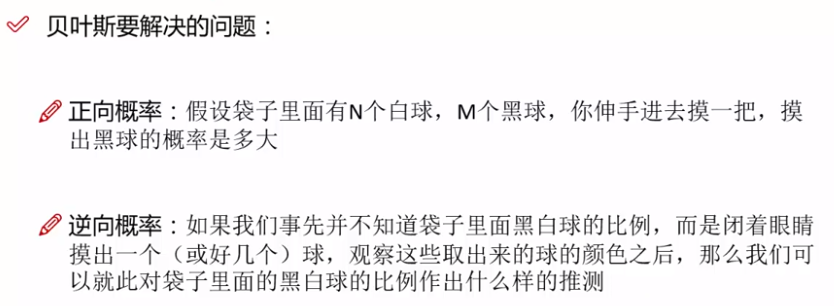### 2、贝叶斯公式

条件概率公式：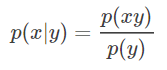全概率公式：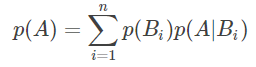贝叶斯公式：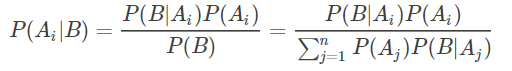其中：p(w)：为先验概率，表示每种类别分布的概率；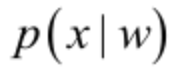：类条件概率，表示在某种类别前提下，某事发生的概率；而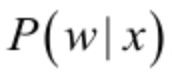为后验概率，表示某事发生了，并且它属于某一类别的概率；p(x)为全概率。

有了这个后验概率，我们就可以对样本进行分类，后验概率越大，说明某事物属于这个类别的可能性越大，我们越有理由把它归到这个类别下。

### 3、案例：

已知：在夏季，某公园男性穿凉鞋的概率为1/2，女性穿凉鞋的概率为2/3，并且该公园中男女比例通常为2:1

问题：若你在公园中随机遇到一个穿凉鞋的人，请问他的性别为男性或女性的概率分别为多少？（已知先验概率，求后验概率问题）

设：w1=男性、w2=女性、x=穿凉鞋

由已知可得到：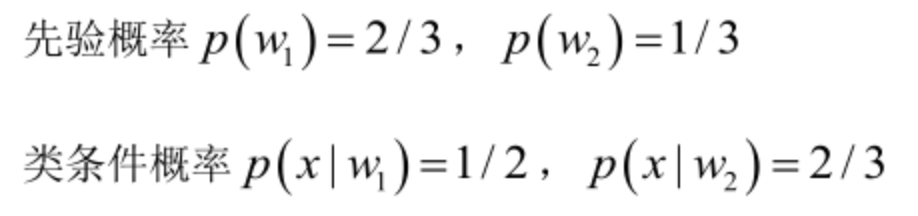男性和女性穿凉鞋相互独立，所以 ：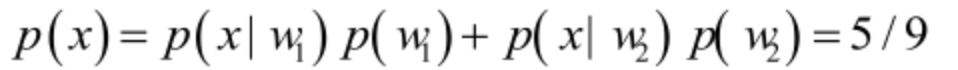（全概率公式）

由贝叶斯公式，可得到：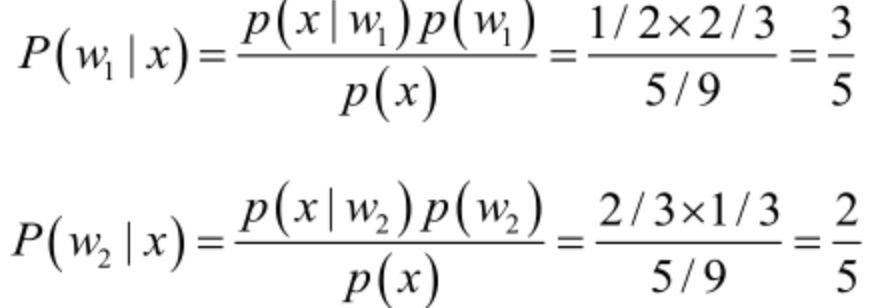展开全文机器学习
• ## 图解贝叶斯公式

千次阅读 2021-03-17 14:35:40
图解贝叶斯公式 文章目录图解贝叶斯公式前言：参考链接：公式背景：以一个例子来理解先验和后验概率：贝叶斯公式：常见名词我的图：总结：联系方式： 前言： 老规矩，先说说为什么要写这篇博客。 研一上《模式识别》...

# 图解贝叶斯公式

## 前言：

老规矩，先说说为什么要写这篇博客。
研一上《模式识别》和《机器学习》的时候，我是弄懂了贝叶斯公式的，当时还觉得这个简单，我理解了。
但是一段时间没用了之后，我自己推导不出来了。
模糊的印象就是，我当时在百度百科上找到了一个非常好的图解例子；
为什么有这样的印象呢？还不是因为百度百科的信息价值一直都比较低，因此偶尔能找到一个靠谱的词条，记忆尤为深刻~

重新走一遍当初的推导过程，并且，记录分享一下~

贝叶斯公式-百度百科

## 公式背景：

贝叶斯定理由英国数学家贝叶斯 ( Thomas Bayes 1702-1761 ) 发展，用来描述两个条件概率之间的关系，比如 P(A|B) 和 P(B|A)。按照乘法法则，可以立刻导出：
P(A∩B) = P(A)*P(B|A)=P(B)*P(A|B)。

这个公式太直观了，事件AB同时发生的概率：
等于当事件A发生了之后，再乘以在事件A发生的基础上事件B发生的概率。
或者事件B发生了之后，再乘以在事件B发生基础上事件A发生的概率。
符合朴素的认知习惯。

如上公式可以稍微变化为：
P(A|B)=P(B|A)*P(A)/P(B)。

这个公式从上往下看也很容易理解，就是等式两端，同除以概率P(B)。
试着直观上描述这个公式。
等式左边是事件B发生后，事件A也被发生的概率；就是下图中间的那个部分P(A∩B)，占整个事件B发生的比例；
等式右边是P(B|A)*P(A)，就是P(B∩A)，也是下图中间的那个部分，除以概率P(B)，也就是占整个B发生的比例。
虽然啰里啰嗦的，但是勉强能把简洁的公式，用语言描述出来了~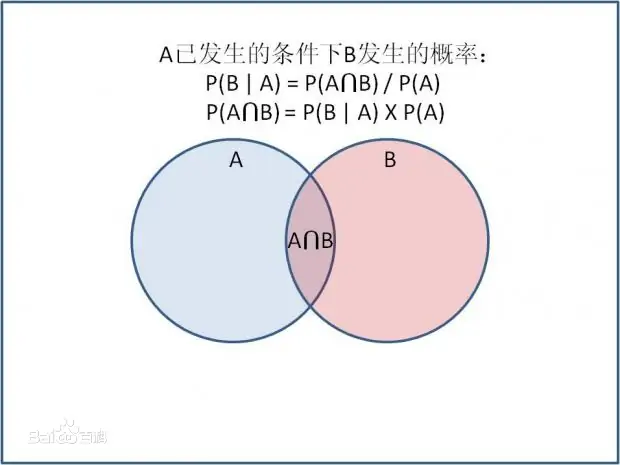## 以一个例子来理解先验和后验概率：

### 贝叶斯公式：

P(A|B)=P(B|A)*P(A)/P(B)

### 常见名词

在贝叶斯定理中，每个名词都有约定俗成的名称：P(A)是 A 的先验概率，之所以称为“先验”是因为它不考虑任何 B 方面的因素。

1. P(A|B)是已知 B 发生后 A 的条件概率，也由于得自 B 的取值而被称作 A 的后验概率
2. P(B|A)是已知 A 发生后 B 的条件概率，也由于得自 A 的取值而被称作 B 的后验概率
3. P(B)是 B 的先验概率，也作标淮化常量（normalizing constant）。

按这些术语，贝叶斯定理可表述为：
后验概率 = (相似度-条件概率 * 先验概率)/标淮化常量

这里面的相似度是第一次出现，其实就是P(B|A)，如果P(B|A)=P(A|B)，那么事件AB是同一个事件，用条件概率当成相似度，倒也貌似合理~

也就是说，后验概率与先验概率和相似度的乘积成正比。
另外，比例P(B|A)/P(B)也有时被称作标淮相似度（standardised likelihood），Bayes定理可表述为：后验概率 = 标淮相似度 * 先验概率

百度百科例子加强版：

现分别有2黑盒子和3个白盒子，在每个黑盒子 里分别有 7 个红球和 3 个白球，在每个白盒子里有1 个红球和 9 个白球。
现已知闭着眼从五个盒子中，摸到了一个盒子，在里面抽出了一个球，是红色的。
问这个红球来自白盒子的概率是多少?

我来写一个较为详细的分析笔记：

在这里例子中，待求的概率是P(从白盒子中|拿到红球)
那么带入公式，可以知道，事件B是拿到了红球，事件A是从白盒子中拿的；
既然明确了事件AB是什么，就从这个题设中，获取已知信息；
P(B|A)=P(拿到红球|从白盒子中)=1/10
P(A)=3/5,因为随机摸盒子，有3/5的概率是白的。
P(B)=P(拿到红球)=3/10，这个就得考虑全盘了，总共红球有5 * 8个，所有的球有5 * 10个
带入可得上面的结果：
P(A│B)=P(B│A)*P(A)/P(B) =1/5

这里的先验概率P(A)是从白盒子中拿，这个和球是什么颜色无关；单纯的看白盒子占所有盒子的中的比例。这个一眼就能看出来，算是先验，应该好理解。

条件概率是P(B|A)，从白盒子中拿到红球的概率；这个就是有条件的了。这个值越大，那么从白盒子中拿红球的概率就越大。

上面两个一乘，就变成了在白盒子中选到红球的概率了

### 我的图：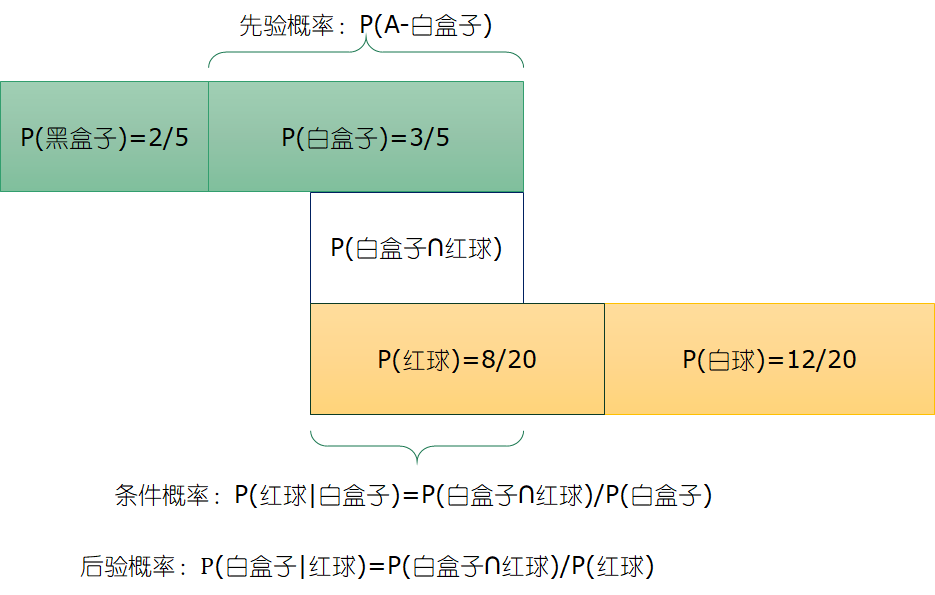图画错了~

标准化常量是P(B)，即拿到红色球的概率。
好好思考一下其中的含义；

## 总结：

贝叶斯公式，用来估计和预测东西还是有点用的。

最后加点其他例子：
贝叶斯公式应用——（1）用来调研尴尬敏感问题

## 联系方式：

ps: 欢迎做强化的同学加群一起学习：

深度强化学习-DRL：799378128

欢迎关注知乎帐号：未入门的炼丹学徒

极简spinup+HER+PER代码实现：https://github.com/kaixindelele/DRLib

展开全文朴素贝叶斯算法
• 通俗地理解贝叶斯公式（定理） 朴素贝叶斯（Naive Bayesian algorithm）是有监督学习的一种分类算法，它基于“贝叶斯定理”实现，该原理的提出人是英国著名数学家托马斯·贝叶斯。贝叶斯定理是基于概率论和统计学的...

## 通俗地理解贝叶斯公式（定理）

朴素贝叶斯（Naive Bayesian algorithm）是有监督学习的一种分类算法，它基于“贝叶斯定理”实现，该原理的提出人是英国著名数学家托马斯·贝叶斯。贝叶斯定理是基于概率论和统计学的相关知识实现的，因此在正式学习“朴素贝叶斯算法”前，我们有必要先认识“贝叶斯定理”。

## 贝叶斯定理

贝叶斯定理的发明者 托马斯·贝叶斯 提出了一个很有意思的假设：“如果一个袋子中共有 10 个球，分别是黑球和白球，但是我们不知道它们之间的比例是怎么样的，现在，仅通过摸出的球的颜色，是否能判断出袋子里面黑白球的比例？”

上述问题可能与我们高中时期所接受的的概率有所冲突，因为你所接触的概率问题可能是这样的：“一个袋子里面有 10 个球，其中 4 个黑球，6 个白球，如果你随机抓取一个球，那么是黑球的概率是多少？”毫无疑问，答案是 0.4。这个问题非常简单，因为我们事先知道了袋子里面黑球和白球的比例，所以很容易算出摸一个球的概率，但是在某些复杂情况下，我们无法得知“比例”，此时就引出了贝叶斯提出的问题。

在统计学中有两个较大的分支：一个是“频率”，另一个便是“贝叶斯”，它们都有各自庞大的知识体系，而“贝叶斯”主要利用了“相关性”一词。下面以通俗易懂的方式描述一下“贝叶斯定理”：通常，事件 A 在事件 B 发生的条件下与事件 B 在事件 A 发生的条件下，它们两者的概率并不相同，但是它们两者之间存在一定的相关性，并具有以下公式（称之为“贝叶斯公式”）：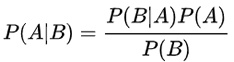看到上述公式，你可能一头雾水，不过不必慌张，下面我们来了解一下“贝叶斯”公式。
符号意义
首先我们要了解上述公式中符号的意义：

P(A) 这是概率中最基本的符号，表示 A 出现的概率。比如在投掷骰子时，P(2) 指的是骰子出现数字“2”的概率，这个概率是 六分之一。

P(B|A) 是条件概率的符号，表示事件 A 发生的条件下，事件 B 发生的概率，条件概率是“贝叶斯公式”的关键所在，它也被称为“似然度”。

P(A|B) 是条件概率的符号，表示事件 B 发生的条件下，事件 A 发生的概率，这个计算结果也被称为“后验概率”。

有上述描述可知，贝叶斯公式可以预测事件发生的概率，两个本来相互独立的事件，发生了某种“相关性”，此时就可以通过“贝叶斯公式”实现预测。

## 条件概率

条件概率是“贝叶斯公式”的关键所在，那么如何理解条件概率呢？其实我们可以从“相关性”这一词语出发。举一个简单的例子，比如小明和小红是同班同学，他们各自准时回家的概率是 P(小明回家) = 1/2 和 P(小红回家) =1/2，但是假如小明和小红是好朋友，每天都会一起回家，那么 P(小红回家|小明回家) = 1 (理想状态下)。

上述示例就是条件概率的应用，小红和小明之间产生了某种关联性，本来俩个相互独立的事件，变得不再独立。但是还有一种情况，比如小亮每天准时到家 P(小亮回家) =1/2，但是小亮喜欢独来独往，如果问 P(小亮回家|小红回家) 的概率是多少呢？你会发现这两者之间不存在“相关性”，小红是否到家，不会影响小亮的概率结果，因此小亮准时到家的概率仍然是 1/2。

贝叶斯公式的核心是“条件概率”，譬如 P(B|A)，就表示当 A 发生时，B 发生的概率，如果P(B|A)的值越大，说明一旦发生了 A，B 就越可能发生。两者可能存在较高的相关性。

## 先验概率

在贝叶斯看来，世界并非静止不动的，而是动态和相对的，他希望利用已知经验来进行判断，那么如何用经验进行判断呢？这里就必须要提到“先验”和“后验”这两个词语。我们先讲解“先验”，其实“先验”就相当于“未卜先知”，在事情即将发生之前，做一个概率预判。比如从远处驶来了一辆车，是轿车的概率是 45%，是货车的概率是 35%，是大客车的概率是 20%，在你没有看清之前基本靠猜，此时，我们把这个概率就叫做“先验概率”。

## 后验概率

在理解了“先验概率”的基础上，我们来研究一下什么是“后验概率？”

我们知道每一个事物都有自己的特征，比如前面所说的轿车、货车、客车，它们都有着各自不同的特征，距离过远的时候，我们无法用肉眼分辨，而当距离达到一定范围内就可以根据各自的特征再次做出概率预判，这就是后验概率。比如轿车的速度相比于另外两者更快可以记做 P(轿车|速度快) = 55%，而客车体型可能更大，可以记做 P(客车|体型大) = 35%。

如果用条件概率来表述 P(体型大|客车)=35%，这种通过“车辆类别”推算出“类别特征”发生的的概率的方法叫作“似然度”。这里的似然就是“可能性”的意思。

## 朴素+贝叶斯

了解完上述概念，你可能对贝叶斯定理有了一个基本的认识，实际上贝叶斯定理就是求解后验概率的过程，而核心方法是通过似然度预测后验概率，通过不断提高似然度，自然也就达到了提高后验概率的目的。

我们知道“朴素贝叶斯算法”由两个词语组成。朴素（native）是用来修饰“贝叶斯”这个名词的。按照中文的理解“朴素”意味着简单不奢华。朴素的英文是“native”，意味着“单纯天真”。

朴素贝叶斯是一种简单的贝叶斯算法，因为贝叶斯定理涉及到了概率学、统计学，其应用相对复杂，因此我们只能以简单的方式使用它，比如天真的认为，所有事物之间的特征都是相互独立的，彼此互不影响。关于朴素贝爷斯算法在下一节会详细介绍。

展开全文深度学习 python
• 下面总结几个我在学习贝叶斯公式的时候能够对我的理解有所帮助的要点，首先引用一句话： “概率论只不过把常识用数学公式表达了出来”——拉普拉斯 贝叶斯公式将人的思维方式用数学公式表达出来，所以贝叶斯公式在...
• 贝叶斯公式 学习机器学习和模式识别的人一定都听过贝叶斯公式(Bayes’ Theorem)： P ( A ∣ B ) = P ( B ∣ A ) P ( A ) P ( B ) P(A|B) = \frac{P(B|A)P(A)}{P(B)}P(A∣B)= P(B) P(B∣A)P(A) ​ 【式1】 贝叶斯公式...机器学习
• ## 贝叶斯公式整理

千次阅读 2020-04-30 19:46:07
1.贝叶斯公式思想 2.事件的贝叶斯公式 3.连续型二维随机变量的贝叶斯公式 4.一般的贝叶斯公式
• 贝叶斯公式的形式化表达为： 公式中，事件Bi的概率为P(Bi)，事件Bi已发生条件下事件A的概率为P(A│Bi)，事件A发生条件下事件Bi的概率为P(Bi│A)。 下面通过一个例子，以通俗易懂的形式说明，贝叶斯公式到底说了...机器学习
• 贝叶斯理论是深度学习机器学习领域中重要的一个理论支撑，这个文档是不错的参考。
• 全概率公式与贝叶斯公式全概率公式贝叶斯公式Reference 全概率公式 给定有限或无限个事件B1,B2,…B_1,B_2,\ldotsB1​,B2​,…，两两互斥且每次试验至少发生一个，即BiBj=∅(i≠j)B_iB_j=\emptyset\left(i\neq j\...
•机器学习 算法
• 目录1 数学上证明贝叶斯公式1.1 预备知识1.2 数学证明2 贝叶斯公式直观理解2.1 问题提出2.2 贝叶斯公式的核心2.3 贝叶斯公式使用情况及计算方式2.3.1 使用情况2.3.2 计算方式3 贝叶斯公式启发4 参考 1 数学上证明...人工智能
• 贝叶斯公式 理论分析 分析 问题 结论 决策判据 应用 【为了证明上帝的存在，他发明了概率统计学原理，遗憾的是，他的这一美好愿望至死也未能实现】 贝叶斯(约1701-1761) Thomas Baye...
• 贝叶斯统计是上述贝叶斯公式的一个简单推广。这个推广，简单地讲，无非是做了下面的一点置换：       进一步，按统计学的习惯写法，将   记作   , 也就是在给定数据下的似然...
• ②全概率公式 ： 在计算一个比较复杂事件的概率时，我们总是希望从已知的简单地事件的概率来计算，为此经常把一个复杂事件分解为若干个不相容的简单事件的和，再分别计算这些简单事件的概率，最后利用有限可加性得到...机器学习 算法
• 贝叶斯分析是整个机器学习的基础框架 中学课本里说概率这个东西表述是一件事情发生的频率， 或者说这叫做客观概率。 贝叶斯框架下的概率理论确从另一个角度给我们展开了答案， 它认为概率是我们个人的一个主观概念，......